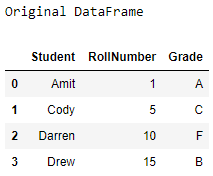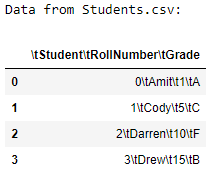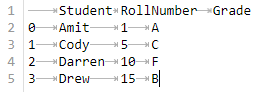# Pandas – DataFrame to CSV file using tab separator

• Last Updated : 27 Aug, 2021

Let’s see how to convert a DataFrame to a CSV file using the tab separator. We will be using the to_csv() method to save a DataFrame as a csv file. To save the DataFrame with tab separators, we have to pass “\t” as the sep parameter in the to_csv() method.

Approach :

1. Import the Pandas and Numpy modules.
2. Create a DataFrame using the DataFrame() method.
3. Save the DataFrame as a csv file using the to_csv() method with the parameter sep as “\t”.
4. Load the newly created CSV file using the read_csv() method as a DataFrame.
5. Display the new DataFrame.

## Python3

 `# importing the modules``import` `pandas as pd``import` `numpy as np` `# creating a DataFrame``students ``=` `{``'Student'``: [``'Amit'``, ``'Cody'``,``                        ``'Darren'``, ``'Drew'``],``            ``'RollNumber'``: [``1``, ``5``, ``10``, ``15``],``            ``'Grade'``: [``'A'``, ``'C'``, ``'F'``, ``'B'``]}``df ``=` `pd.DataFrame(students,``                  ``columns ``=``[``'Student'``, ``'RollNumber'``,``                            ``'Grade'``])``# displaying the original DataFrame``print``(``"Original DataFrame"``)``print``(df)` `# saving as a CSV file``df.to_csv(``'Students.csv'``, sep ``=``'\t'``)` `# loading the CSV file``new_df ``=` `pd.read_csv(``'Students.csv'``)` `# displaying the new DataFrame``print``(``'Data from Students.csv:'``)``print``(new_df)`

Output :The contents of the Students.csv file are :My Personal Notes arrow_drop_up